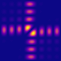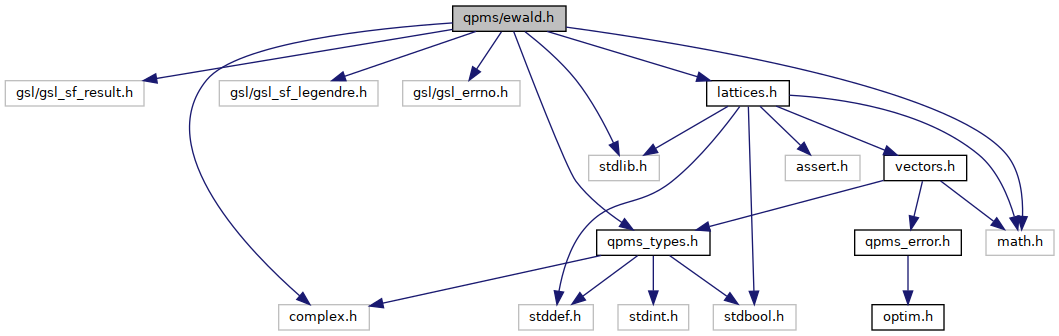QPMS Electromagnetic multiple scattering library and toolkit.
ewald.h File Reference

Lattice sums of spherical waves. More...

#include <gsl/gsl_sf_result.h>
#include <stdlib.h>
#include <gsl/gsl_sf_legendre.h>
#include <gsl/gsl_errno.h>
#include <math.h>
#include <complex.h>
#include "qpms_types.h"
#include "lattices.h"
Include dependency graph for ewald.h:Go to the source code of this file.

## Data Structures

struct  qpms_ewald3_constants_t
Object holding the Ewald sum constant factors. More...

struct  qpms_csf_result
Structure for holding complex-valued result of computation and an error estimate. More...

## Typedefs

typedef struct qpms_ewald3_constants_t qpms_ewald3_constants_t
Object holding the Ewald sum constant factors. More...

typedef struct qpms_csf_result qpms_csf_result
Structure for holding complex-valued result of computation and an error estimate. More...

## Enumerations

enum  qpms_ewald_part { QPMS_EWALD_LONG_RANGE = 1 , QPMS_EWALD_SHORT_RANGE = 2 , QPMS_EWALD_0TERM = 4 , QPMS_EWALD_FULL = QPMS_EWALD_LONG_RANGE | QPMS_EWALD_SHORT_RANGE | QPMS_EWALD_0TERM }

## Functions

qpms_ewald3_constants_tqpms_ewald3_constants_init (qpms_l_t lMax, int csphase)
Constructor for qpms_ewald3_constants_t.

void qpms_ewald3_constants_free (qpms_ewald3_constants_t *)
Destructor for qpms_ewald3_constants_t.

static complex double lilgamma (double t)

static complex double clilgamma (complex double z)

int cx_gamma_inc_series_e (double a, complex double z, qpms_csf_result *result)
Incomplete Gamma function as a series. More...

int cx_gamma_inc_CF_e (double a, complex double z, qpms_csf_result *result)
Incomplete Gamma function as continued fractions. More...

int complex_gamma_inc_e (double a, complex double x, int m, qpms_csf_result *result)
Incomplete gamma for complex second argument. More...

int complex_expint_n_e (int n, complex double x, qpms_csf_result *result)
Exponential integral for complex second argument. More...

int hyperg_2F2_series (double a, double b, double c, double d, double x, gsl_sf_result *result)
Hypergeometric 2F2, used to calculate some errors.

void ewald3_2_sigma_long_Delta (complex double *target, double *target_err, int maxn, complex double x, int xbranch, complex double z)
The Delta_n factor from [Kambe II], Appendix 3, used in 2D-in-3D long range sum. More...

void ewald3_2_sigma_long_Delta_recurrent (complex double *target, double *target_err, int maxn, complex double x, int xbranch, complex double z, _Bool bigimz)
The Delta_n factor from [Kambe II], Appendix 3, used in 2D-in-3D long range sum. More...

void ewald3_2_sigma_long_Delta_series (complex double *target, double *target_err, int maxn, complex double x, int xbranch, complex double z)
The Delta_n factor from [Kambe II], Appendix 3, used in 2D-in-3D long range sum. More...

int ewald3_sigma0 (complex double *result, double *err, const qpms_ewald3_constants_t *c, double eta, complex double wavenumber)
The Ewald sum "self-interaction" term that appears in the lattice sums with zero (direct-space) Bravais lattice displacement. More...

int ewald3_sigma_short (complex double *target_sigmasr_y, double *target_sigmasr_y_err, const qpms_ewald3_constants_t *c, double eta, complex double wavenumber, LatticeDimensionality latdim, PGen *pgen_R, bool pgen_generates_shifted_points, cart3_t k, cart3_t particle_shift)
Short-range part of outgoing scalar spherical wavefunctions' lattice sum $$\sigma_{l,m}^\mathrm{S}(\vect k,\vect s)$$. More...

int ewald3_sigma_long (complex double *target_sigmalr_y, double *target_sigmalr_y_err, const qpms_ewald3_constants_t *c, double eta, complex double wavenumber, double unitcell_volume, LatticeDimensionality latdim, PGen *pgen_K, bool pgen_generates_shifted_points, cart3_t k, cart3_t particle_shift)
Long-range part of outgoing scalar spherical wavefunctions' lattice sum $$\sigma_{l,m}^\mathrm{L}(\vect k,\vect s)$$. More...

## Variables

gsl_error_handler_t IgnoreUnderflowsGSLErrorHandler
Use this handler to ignore underflows of incomplete gamma.

## Detailed Description

Lattice sums of spherical waves.

Implementation of two-dimensional lattice sum in three dimensions according to:

•  C.M. Linton, I. Thompson Journal of Computational Physics 228 (2009) 1815–1829
•  C.M.Linton SIAM Review Vol 52, No. 4, pp. 630–674

N.B.!!! currently, the long-range parts are calculated not according to [1,(4.5)], but rather according to the spherical-harmonic-normalisation-independent formulation in my notes notes/ewald.lyx. Both parts of lattice sums are then calculated with the $$P_n^{|m|} e^{im\phi}$$ (N.B. or $$P_n^{|m|} e^{imf} (-1)^m$$ for negative m) substituted in place of $$Y_n^m$$ (this is quite a weird normalisation especially for negative $$|m|$$, but it is consistent with the current implementation of the translation coefficients in translations.c; in the long run, it might make more sense to replace it everywhere with normalised Legendre polynomials).

## ◆ qpms_csf_result

 typedef struct qpms_csf_result qpms_csf_result

Structure for holding complex-valued result of computation and an error estimate.

Similar to gsl_sf_result, but with complex val.

## ◆ qpms_ewald3_constants_t

 typedef struct qpms_ewald3_constants_t qpms_ewald3_constants_t

Object holding the Ewald sum constant factors.

Used internally by qpms_translation_calculator_t. Initialised by qpms_ewald3_constants_init() and freed by qpms_ewald3_constants_free().

## ◆ complex_expint_n_e()

 int complex_expint_n_e ( int n, complex double x, qpms_csf_result * result )

Exponential integral for complex second argument.

If x is (almost) positive real, it just uses gsl_sf_expint_En_e().

## ◆ complex_gamma_inc_e()

 int complex_gamma_inc_e ( double a, complex double x, int m, qpms_csf_result * result )

Incomplete gamma for complex second argument.

If x is (almost) real, it just uses gsl_sf_gamma_inc_e().

On the negative real axis (where the function has branch cut), the sign of the imaginary part is what matters (even if it is zero). Therefore one can have complex_gamma_inc_e(a, z1, m) != complex_gamma_inc_e(a, z2, m) even if z1 == z2, because -0 == 0 according to IEEE 754. The side of the branch cut can be determined using signbit(creal(z)).

Another than principal branch can be selected using non-zero m argument.

Parameters
 m Branch index. If zero, the principal value is calculated. Other branches might be chosen using non-zero m. In such case, the returned value corresponds to $\Gamma(a,ze^{2\pi mi})=e^{2\pi mia} \Gamma(a,z) + (1-e^{2\pi mia}) \Gamma(a).$

If a is non-positive integer, the limiting value should be used, but this is not yet implemented!

## ◆ cx_gamma_inc_CF_e()

 int cx_gamma_inc_CF_e ( double a, complex double z, qpms_csf_result * result )

Incomplete Gamma function as continued fractions.

The principal value is calculated. On the negative real axis (where the function has branch cut), the sign of the imaginary part is what matters (even if it is zero). Therefore one can have cx_gamma_inc_CF_e(a, z1) != cx_gamma_inc_CF_e(a, z2) even if z1 == z2, because -0 == 0 according to IEEE 754. The side of the branch cut can be determined using signbit(creal(z)).

## ◆ cx_gamma_inc_series_e()

 int cx_gamma_inc_series_e ( double a, complex double z, qpms_csf_result * result )

Incomplete Gamma function as a series.

DLMF 8.7.3 (latter expression) for complex second argument.

The principal value is calculated. On the negative real axis (where the function has branch cut), the sign of the imaginary part is what matters (even if it is zero). Therefore one can have cx_gamma_inc_series_e(a, z1) != cx_gamma_inc_series_e(a, z2) even if z1 == z2, because -0 == 0 according to IEEE 754. The side of the branch cut can be determined using signbit(creal(z)).

## ◆ ewald3_2_sigma_long_Delta()

 void ewald3_2_sigma_long_Delta ( complex double * target, double * target_err, int maxn, complex double x, int xbranch, complex double z )

The Delta_n factor from [Kambe II], Appendix 3, used in 2D-in-3D long range sum.

$\Delta_n = \int_n^\infty t^{-1/2 - n} \exp(-t + z^2/(4t))\ud t$

Bug:
The current choice of method, based purely on the value of z, might be unsuitable especially for big values of maxn.

## ◆ ewald3_2_sigma_long_Delta_recurrent()

 void ewald3_2_sigma_long_Delta_recurrent ( complex double * target, double * target_err, int maxn, complex double x, int xbranch, complex double z, _Bool bigimz )

The Delta_n factor from [Kambe II], Appendix 3, used in 2D-in-3D long range sum.

This function always uses Kambe's (corrected) recurrent formula. For production, use ewald3_2_sigma_long_Delta() instead.

## ◆ ewald3_2_sigma_long_Delta_series()

 void ewald3_2_sigma_long_Delta_series ( complex double * target, double * target_err, int maxn, complex double x, int xbranch, complex double z )

The Delta_n factor from [Kambe II], Appendix 3, used in 2D-in-3D long range sum.

This function always uses Taylor expansion in z. For production, use ewald3_2_sigma_long_Delta() instead.

Bug:
The error estimate seems to be wrong (too small) at least in some cases: try parameters maxn = 40, z = 0.5, x = -3. This might be related to the exponential growth of the error.

## ◆ ewald3_sigma0()

 int ewald3_sigma0 ( complex double * result, double * err, const qpms_ewald3_constants_t * c, double eta, complex double wavenumber )

The Ewald sum "self-interaction" term that appears in the lattice sums with zero (direct-space) Bravais lattice displacement.

Parameters
 result Pointer to save the result (single complex double). err Pointer to save the result error estimate (single double). c Constant factors structure initialised by qpms_ewald3_constants_init(). eta Ewald parameter. wavenumber Wavenumber of the background medium.

## ◆ ewald3_sigma_long()

 int ewald3_sigma_long ( complex double * target_sigmalr_y, double * target_sigmalr_y_err, const qpms_ewald3_constants_t * c, double eta, complex double wavenumber, double unitcell_volume, LatticeDimensionality latdim, PGen * pgen_K, bool pgen_generates_shifted_points, cart3_t k, cart3_t particle_shift )

Long-range part of outgoing scalar spherical wavefunctions' lattice sum $$\sigma_{l,m}^\mathrm{L}(\vect k,\vect s)$$.

Parameters
 target_sigmalr_y Target array for $$\sigma_{l,m}^\mathrm{L}$$, must be c->nelem_sc long. target_sigmalr_y_err Target array for error estimates, must be c->nelem_sc long or NULL. c Constant factors structure initialised by qpms_ewald3_constants_init(). eta Ewald parameter. wavenumber Wavenumber of the background medium. unitcell_volume Volume of the (direct lattice) unit cell (with dimension corresponding to the lattice dimensionality). latdim Lattice dimensionality. pgen_K Lattice point generator for the reciprocal lattice. There is a possibility that the whole PGen is not consumed (this might happen if the summand start to be consistently smaller than the (partial) sums * DBL_EPSILON. In such case, it is the responsibility of the caller to deallocate the generator. pgen_generates_shifted_points Indicates whether pgen_K already generates shifted points. If false, the behaviour corresponds to the old ewald32_sigma_long_points_and_shift(), so the function assumes that the generated points correspond to the unshifted reciprocal Bravais lattice, and adds beta to the generated points before calculations. If true, it assumes that they are already shifted. k Wave vector $$\vect k$$. particle_shift Lattice offset $$\vect s$$ wrt. the Bravais lattice.

## ◆ ewald3_sigma_short()

 int ewald3_sigma_short ( complex double * target_sigmasr_y, double * target_sigmasr_y_err, const qpms_ewald3_constants_t * c, double eta, complex double wavenumber, LatticeDimensionality latdim, PGen * pgen_R, bool pgen_generates_shifted_points, cart3_t k, cart3_t particle_shift )

Short-range part of outgoing scalar spherical wavefunctions' lattice sum $$\sigma_{l,m}^\mathrm{S}(\vect k,\vect s)$$.

Parameters
 target_sigmasr_y Target array for $$\sigma_{l,m}^\mathrm{S}$$, must be c->nelem_sc long. target_sigmasr_y_err Target array for error estimates, must be c->nelem_sc long or NULL. c Constant factors structure initialised by qpms_ewald3_constants_init(). eta Ewald parameter. wavenumber Wavenumber of the background medium. latdim Lattice dimensionality. Ignored apart from asserts and possible optimisations, as the SR formula stays the same. pgen_R Lattice point generator for the direct Bravais lattice. There is a possibility that the whole PGen is not consumed (this might happen if the summand start to be consistently smaller than the (partial) sums * DBL_EPSILON. In such case, it is the responsibility of the caller to deallocate the generator. pgen_generates_shifted_points Indicates whether pgen_R already generates shifted points. If false, the behaviour corresponds to the old ewald32_sigma_short_points_and_shift(), so the function assumes that the generated points correspond to the unshifted Bravais lattice, and adds particle_shift to the generated points before calculations. If true, it assumes that they are already shifted (if calculating interaction between different particles in the unit cell). k Wave vector $$\vect k$$. particle_shift Lattice offset $$\vect s$$ wrt. the Bravais lattice.